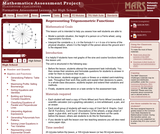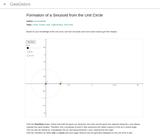# 2 Results

View
Selected filters:
• Unit CirclesOnly Sharing Permitted
CC BY-NC-ND
Rating

This lesson unit is intended to help teachers assess how well students are able to: model a periodic situation, the height of a person on a Ferris wheel, using trigonometric functions; and interpret the constants a, b, c in the formula h = a + b cos ct in terms of the physical situation, where h is the height of the person above the ground and t is the elapsed time.

Material Type:
Assessment
Lesson Plan
Provider:
Shell Center for Mathematical Education
U.C. Berkeley
Provider Set:
Mathematics Assessment Project (MAP)
04/26/2013Conditional Remix & Share Permitted
CC BY-SA
Rating

Based on knowledge of the unit Circles, students see how sinusoids (Sine and coSine waves) get their shapes.

Material Type:
Simulation
Provider:
GeoGebra
Provider Set:
GeoGebraTube